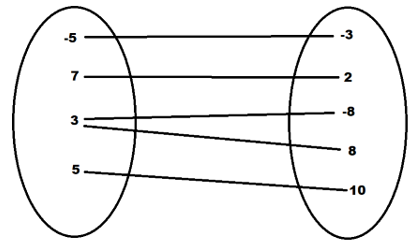Maths-
General
Easy

Question

# Does the relation { (-5,-3)(7,2)(3,8)(3,-8)(5,10)} represent a function ? Use the arrow diagram . Then explain your answerHint:

## The correct answer is: {-3 , 2 , 8 ,-8 ,10}

### We have given the set of ordered pairs { (-5,-3)(7,2)(3,8)(3,-8)(5,10)}Domain :- {-5, 7 , 3, 5}Co- domain :- {-3 , 2 , 8 ,-8 ,10}a) Arrow diagram of the given data isb) From the given data we can analyse that the member in the domain set i.e. 3 has two images i.e. 8 and -8 in the co-domainWe know that in a function one member in the domain set cannot have two images in the co-domain set.Therefore, the given relation is not a function.

All functions are relations but all relations are not functions.#### With Turito Foundation.#### Get an Expert Advice From Turito.